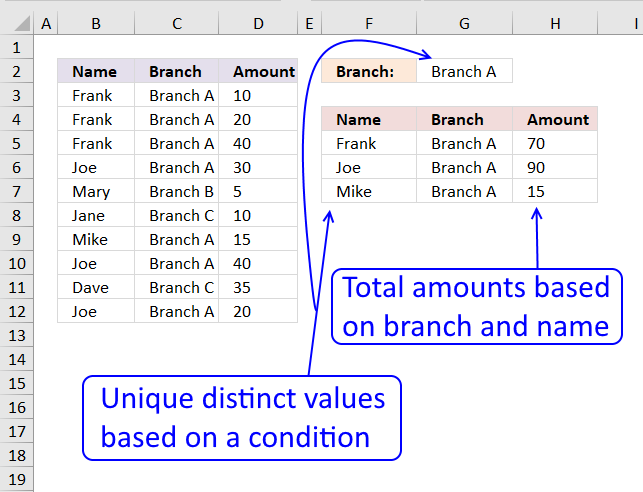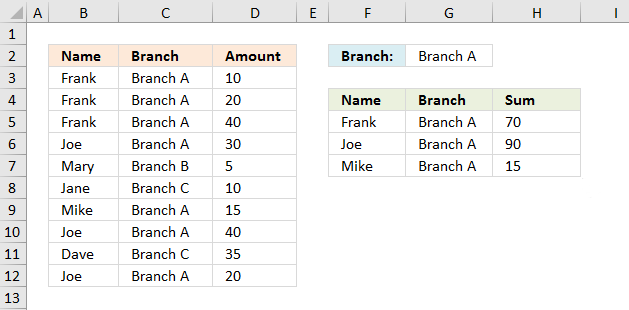Author: Oscar Cronquist Article last updated on February 18, 2018Is it possible to extend this by matching items that meet a criteria?

I have a list of credit card transactions showing the name of the card holder, their Branch and the amount. I want to produce a report for each Branch, so what I want is to extract only those people who match the Branch name. For example:

Frank Branch A
Frank Branch A
Frank Branch A
Joe Branch A
Mary Branch B
Jane Branch C
Mike Branch A
Joe Branch A
Dave Branch C

I would like a list of only those people for Branch A, and then be able to summarise the transactions. Or should I do this in two stages?

This post demonstrates how to build a formula to extract a unique distinct list using a condition, however, I highly recommend a pivot table for this task: Pivot table - Unique distinct listType a branch in cell G2 and the formulas in cell range F5:H7 returns the correct records from B3:D12. If you have more than two values in one record (except the number) use the COUNTIFS function to filter unique distinct records.

Array formula in F5:

=INDEX(\$B\$3:\$D\$11, MATCH(0, COUNTIF(\$E\$7:\$E7, \$A\$2:\$A\$11)+(\$B\$2:\$B\$11<>\$F\$1), 0), COLUMN(A1))

Copy cell F5 and paste to cell range F5:G7.

Formula in cell H5:

=SUMIFS(\$D\$3:\$D\$12, \$B\$3:\$B\$12, F5, \$C\$3:\$C\$12, C3)

If you have a version earlier than Excel 2007 use the SUMPRODUCT function

=SUMPRODUCT((F5=\$B\$3:\$B\$12)*(G5=\$C\$3:\$C\$12)*\$D\$3:\$D\$12)

If you are interested in how the SUMPRODUCT function works, read:

How to use the SUMPRODUCT function

The SUMPRODUCT function calculates the product of corresponding values and then returns the sum of each multiplication.

### Watch a video where I explain the formula

https://youtu.be/FrGofo7NCVo

5 easy ways to extract Unique Distinct Values

First, let me explain the difference between unique values and unique distinct values, it is important you know the difference […]

### How to enter an array formula

1. Copy (Ctrl + c) above formula
2. Double click on cell E8
3. Paste (Ctrl + v) to cell
4. Press and hold CTRL + Shift simultaneously
5. Press Enter
6. Release all keys

Copy cell E8 and paste it down as far as needed. Copy cells and paste into cell range F8 and down as far as needed.

How to enter an array formula

Array formulas allows you to do advanced calculations not possible with regular formulas.

### Explaining array formula in cell E8

Step 1 - Check previous values to make sure they are not repeated

COUNTIF(\$E\$7:\$E7, \$A\$2:\$A\$11)

If you examine the cell references you may find this strange: \$E\$7:\$E7 It is an absolute and relative cell reference. It expands when the formula is copied across the spreadsheet. Read more here: Absolute and relative references in excel

COUNTIF(\$E\$7:\$E7, \$A\$2:\$A\$11)

becomes

COUNTIF("Name", {"Frank"; "Frank"; "Frank"; "Joe"; "Mary"; "Jane"; "Mike"; "Joe"; "Dave"; "Joe"})

and returns

{0;0;0;0;0;0;0;0;0;0}

Recommended article

How to use the COUNTIF function

Counts the number of cells that meet a specific condition.

Step 2 - Compare values in column B with the value in cell F1

(\$B\$2:\$B\$11<>\$F\$1)

becomes

{"Branch A"; "Branch A"; "Branch A"; "Branch A"; "Branch B"; "Branch C"; "Branch A"; "Branch A"; "Branch C"; "Branch A"}<>"Branch A"

and returns {FALSE; FALSE; FALSE; FALSE; TRUE; TRUE; FALSE; FALSE; TRUE; FALSE}

COUNTIF(\$E\$7:\$E7, \$A\$2:\$A\$11)+(\$B\$2:\$B\$11<>\$F\$1)

becomes

{0;0;0;0;0;0;0;0;0;0} + {FALSE; FALSE; FALSE; FALSE; TRUE; TRUE; FALSE; FALSE; TRUE; FALSE}

and returns

{0;0;0;0;1;1;0;0;1;0}

Step 4 - Find first 0 (zero) in array

MATCH(0, COUNTIF(\$E\$7:\$E7, \$A\$2:\$A\$11)+(\$B\$2:\$B\$11<>\$F\$1), 0)

becomes

MATCH(0, {0;0;0;0;1;1;0;0;1;0}, 0)

and returns 1.

Recommended article

How to use the MATCH function

Identify the position of a value in an array.

Step 5 - Return a value or reference of the cell at the intersection of a particular row and column

=INDEX(\$A\$2:\$C\$11, MATCH(0, COUNTIF(\$E\$7:\$E7, \$A\$2:\$A\$11)+(\$B\$2:\$B\$11<>\$F\$1), 0), COLUMN(A1))

becomes

=INDEX(\$A\$2:\$C\$11, 1, COLUMN(A1))

becomes

=INDEX(\$A\$2:\$C\$11, 1, 1)

becomes

=INDEX({"Frank","Branch A",10; "Frank","Branch A",20; "Frank","Branch A",40; "Joe","Branch A",30; "Mary","Branch B",5; "Jane","Branch C",10; "Mike","Branch A",15; "Joe","Branch A",40; "Dave","Branch C",35; "Joe","Branch A",20}, 1, 1)

and returns "Frank" in cell E8.

How to use the INDEX function

Gets a value in a specific cell range based on a row and column number.

unique distinct list matching criteria.xls
(Excel 97-2003 Workbook *.xls)

Recommended articles:

Filter unique distinct records with a condition

Sean asks: If Tea and Coffee has Americano,it will only return Americano once and not twice. I am looking for a […]

Filter unique distinct values where adjacent cells contain search string

Question: How do I create a unique distinct list where adjacent cell values contain a search string? AA102 CA AA103 […]

Extract unique distinct values sorted based on sum of adjacent values

Filtering unique distinct text values and sort them based on the sum of adjacent values can easily be done by […]

IF(logical_test,[value_if:true];[value_if_false])
Checks whether a condition is met, and returns one value if TRUE, and another value if FALSE

SMALL(array,k)
Returns the k-th smallest number in this data set.

ROW(reference)
Returns the rownumber of a reference

INDEX(array,row_num,[column_num])
Returns a value or reference of the cell at the intersection of a particular row and column, in a given range

MATCH(lookup_value;lookup_array, [match_type])
Returns the relative position of an item in an array that matches a specified value

COUNTIF(range,criteria)
Counts the number of cells within a range that meet the given condition

SUMPRODUCT(array1, array2, )
Returns the sum of the products of the corresponding ranges or arrays